# Canvas Rectangle in HTML5

### Canvas rectangles

Rectangles are used to create the rectangle by using properties of the rectangle

Drawing rectangles by using `fillRect` or `strokeRect` methods.

```    <canvas id="myCanvas" width="578" height="250"></canvas>

<br />
<input type="button" onclick="DrawRectangle()" value="Draw Rectangles" />

<script type="text/javascript">
function DrawRectangle() {
var context = document.getElementById('myCanvas').getContext('2d');
context.beginPath();
context.lineWidth = "5";
// rect(x, y, rectangle-width, rectangle-height);
context.strokeRect(10, 10, 50, 100);
context.fillStyle = "Red";
context.fillRect(20, 20, 30, 50);
context.strokeStyle = "green";
context.strokeRect(150, 100, 100, 100);
context.globalAlpha = 0.5;
context.fillStyle = "red";
context.fillRect(200, 150, 100, 100);
}
</script>

```

In the above code snippet, on click of the button, the `DrawRectangle()` function executes.

As usual we got our context object from the canvas and called the beginPath() method to start drawing the rectangle.

The xxxRect method accepts four parameter

• 1st parameter is the x coordinates from where we want to start the rectangle
• 2nd parameter is the y coordinates from where we want to start the rectangle
• 3rd parameter is the width of rectangle we want to draw
• 4th parameter is the height of the rectangle we want to draw

In the beginning itself we have specified the `lineWidth `value so that all rectangle we are going to draw will use the same line width (it is nothing but border width)

##### strokeRect() method

This method starts the drawing the rectangle from 10, 10 (x, y) pixel on the canvas with width as 50 and height as 100 pixel of the rectangle.

##### fillRect() method

This method draw a filled rectangle starting from 20, 20 position (x, y) with width as 30 and height as 50. As before this method we have set the fillStyle to “Red” so this rectangle will be drawn filled with red color. Note that you need to set the fillStyle before calling the `fillRect()` method or the rectangle will be drawn filled with default black color.

Similarly, we have drawn a rectangle with green border by setting the strokeStyle to “green”. To draw a shape with transparent background, we need to set the `globalAlpha `value (0 is full transparent and 1 is opaque).

Output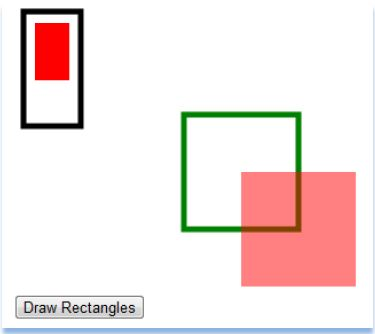### rect()

It is used to create the rectangle by using `rect()` property

```<canvas id="myCanvas" width="300" height="150" style="border:3px dotted black;"></canvas>
<script>
var a = document.getElementById("myCanvas");
var b = a.getContext("2d");
b.rect(40, 40, 200, 100);
b.stroke();
</script>
```

rect()

In the above code snippet we have defined the rectangle using `rect()`. We have taken the `<canvas>` id as `"mycanvas"`  and width as "300" and height as "150" which creates the rectangle with dotted black color and we have taken script to design the rectangle by using javascript. We have taken the `var a` as `document.getElementById` as we declared `id` as `mycanvas` in the canvas element and `var b` as `a.getcontext ("2d")` is a method to create the rectangle and we have given the measurement as `b.rect(40, 40, 200, 100);` which is used to set the height, width, left and right side positions, In the lastline we are having the b.stroke() which is used to draw the path.

output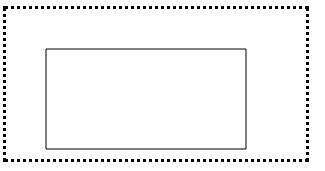### fillrect()

It is used to fill the rectangle with full given properties of the  color

```<canvas id="myCanvas" width="200" height="100" style="border:8px solid blue;"></canvas>
<script>
var a = document.getElementById("myCanvas");
var b = a.getContext("2d");
b.fillRect(40, 20, 50, 100);
</script>
```

fillrect()

In the above code snippet we have defined the `fillrect()` which is used to fill the rectangle with the color. We have taken the `var a as document .getelementById` as "mycanvas" and var b as `a.getcontext("2d")`  which is a method and ` b.fillRect(40, 20, 50, 100)`; is used to print the rectangle by using the values.

output### StrokeRect()

It is used to draw a rectangle with no fill color

```<canvas id="myCanvas" width="200" height="100" style="border:4px solid yellow;"></canvas>
<script>
var a = document.getElementById("myCanvas");
var b = a.getContext("2d");
b.strokeRect(40, 10, 50, 80);
</script>
```

Stroke rect()

In the above code snippet we have defined the `strokerect() `which is used to unfill in the rectangle  We have taken the `var a as document .getelementById` as mycanvas and `var b as a.getcontext("2d") `which is a method and  b.strokeRect(40, 10, 50, 80); which prints the rectangle by using the values.

output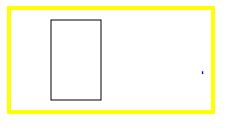### ClearRect():

It is used to clear the rectangle in the webpage

``` <canvas id="myCanvas" width="200" height="100" style="border:4px solid pink;"></canvas>
<script>
var a = document.getElementById("myCanvas");
var b = a.getContext("2d");
b.clearRect(40, 10, 50, 80);
</script>
```

Clearrect()

In the above code snippet we have defined the `ClearRect()` which is used to clear the rectangle  We have taken the `var a as document .getelementById `as "mycanvas" and `var b as a.getcontext("2d")` which is a method and  b.strokeRect(40, 10, 50, 80); which clears the rectangle by using the values.

output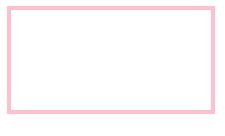### canvas with Rectangular color:

It is used to add the colors using rectangular properties

```<canvas id="myCanvas" width="500" height="400"></canvas>
<script>
var canvas = document.getElementById('myCanvas');
var context = canvas.getContext('2d');
context.beginPath();
context.rect(18, 50, 200, 100);
context.fillStyle = "red";
context.fill();
context.lineWidth = "20";
context.strokeStyle = "pink";
context.stroke();
</script>```

Canvas rectangle

• In the above code snippet we have defined the canvas with rectangle we have taken the <canvas> id as my canvas, we have given the width and height of the canvas and in the script to add the properties of the rectangle we have var canvas as document .getElementById as mycanvas and var context as canvas .getcontext ("2d") which is a method
• context.begin path is used to begin the rectangle
• context.rect(18,50,200,100) is used to add the left, top, width and height.
• context.fillstyle as red is used add the red color to the rectangle
• context.linewidth is used to add the width of the line
• context.strokestyle is used to add the color to the line width with the given color.

output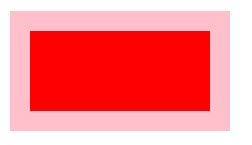### Canvas rectangle with glopha alpha

Canvas rectangle with global alpha is used to change the color of the rectangle

```<canvas id="myCanvas" width="500" height="400"></canvas>
<script>
var canvas = document.getElementById('myCanvas');
var context = canvas.getContext('2d');
context.beginPath();
context.rect(18, 50, 200, 100);
context.fillStyle = "red";
context.globalAlpha = "0.7";
context.fill();
context.lineWidth = "20";
context.strokeStyle = "pink";
context.stroke();
</script>
```

Canvas rectangle with global alpha

In the above code snippet we have defined the global alpha, which is used to increment and decrement the color by using the context.globalalpha="0.7", By incrementing the global alpha from 0.7 it appears as bright and by decrementing the global alpha from "0.7" the brightness of the rectangle decreases

outputCanvas rectangle with onclick function

We can use the canvas rectangle with onclick function by using javascript

``` <canvas id="Canvas1" onclick="color()" style="border: 5px solid green"></canvas>
<script>
var c = document.getElementById("Canvas1");
var b = c.getContext("2d");
b.fillStyle = "yellow";
b.fillRect(100, 30, 80, 90);
function color() {
{
}
}
</script>
```

Canvas rectangle with onclick

In the above code snippet we have taken `onclick` function with javascript function we have taken function a color and we have given onclick function as `color()` which is used as when click function on the yellow color it displays the alert box as `Hello am yellow color`.

outputAfter giving the onclick to the yellow rectangle it will display the alert box as "Hai am yellow color"

outputViews: 12823 | Post Order: 42